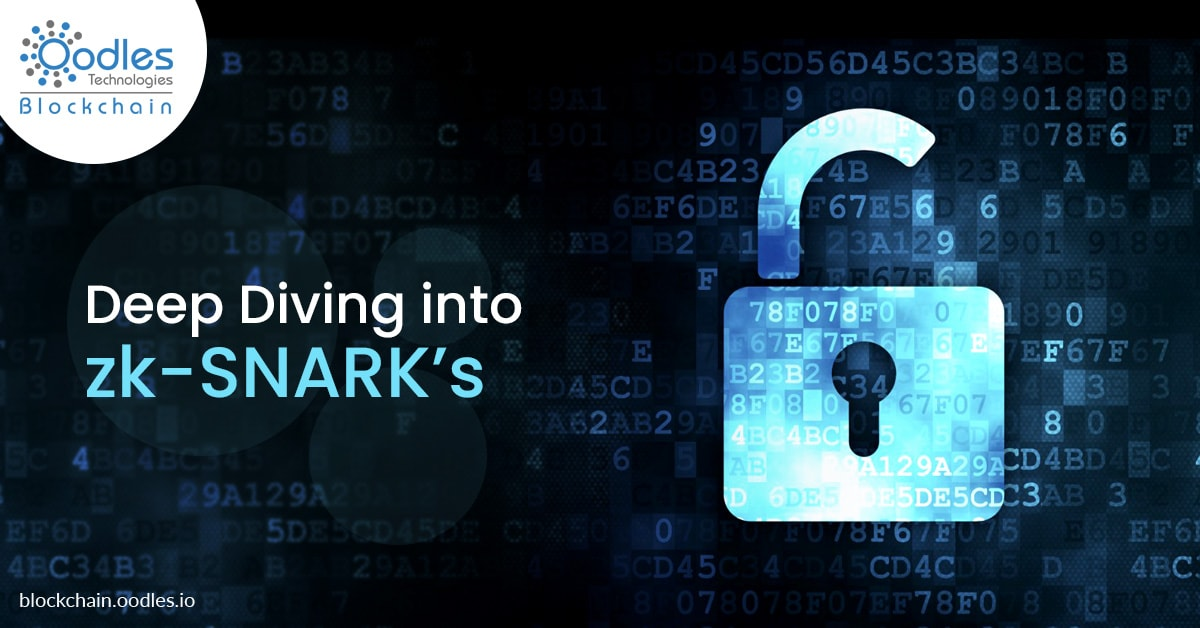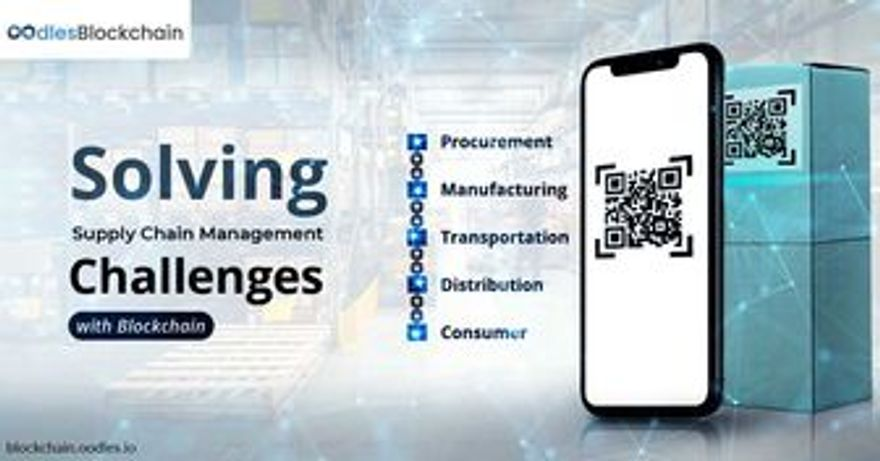zk-Snark Proof | Diving deep into its Technical Aspects• # Deep Diving Into zk-SNARKIn the previous blog “An Introduction to zk-SNARK’s”, we briefly describe what it is and followed it with a use case where and how it can be applied.

In this blog, we will dig into the technical aspects of zk-SNARK. We’ll first see what properties a zk-SNARK proof constitutes and the process involved in the generation of zk-SNARK proof.

There are certain properties a proof must satisfy to be called zk-SNARK, which are listed as below;

Completeness

This property states that “if the statement is true, and the prover and the verifier are trusted (honest), the proof is accepted.”

Soundness

This property states that “if the statement is false, then the cheating prover cannot convince an honest(trusted) verifier that the statement is true except some little probability.”

In addition to the two main properties listed above, a zk-SNARK also needs to be:

Zero Knowledge

This means that the verifier does not learn anything about the statement, apart from being either the statement is true or false.

Succinct

This means that the size of the proof should suffice its size so that it can be proved in milliseconds.

Non-Interactive

This means that there should not be any back and forth communication between a prover and a verifier, to enforce that only one set of essential information is sent to the verifier at once.

Argument

The argument passed for generating a proof must be soundproof, soundness holds against a prover that leverages polynomial time, i.e. it guarantees bounded computation.

Of Knowledge

This means that the proof cannot be constructed without the access to the witness.
Here witness is defined as the private input needed to prove the statement.

All the property listed above constitute a zk-SNARK i.e, Zero-Knowledge Succinct Non-Interactive Argument of Knowledge.

Now, after establishing the properties of what a zk-SNARK proof must constitute, let us examine how these proofs are generated:

To generate a zk-SNARK, it essentially involves processes described as below :

Computation

The very first step involved in the generation of a zk-SNARK is to turn the proving statement into an equivalent Algebraic Expression.
Let us assume that we obtained following algebraic expression (a + b) * b * c

Arithmetic Circuit

The next step is to create an Arithmetic Circuit for the evaluation of computational expression obtained in the first step.
An Arithmetic Circuit is similar to a Boolean Circuit where a program is compiled down to a single discrete step consisting of very basic atomic arithmetic operations such as addition, multiplication etc.

Below is a figure depicting how the Arithmetic Circuit would look like for the expression listed in the first step:

Rank-1 Constraint System (R1CS)

If we look at the above figure, we can assume that the values A, B, and C are traveling in a certain direction, i.e from left to right. As the name suggests an R1CS is responsible for checking whether the value travels correctly or not.

If we see an R1CS, it has to check many constraints, almost one for every wire. Although our version of R1CS is very basic and simple, however in a practical scenario the computed arithmetic expression is much more complex resulting in a complex R1CS, which becomes time-consuming to check.

To minimize this, we can bundle these constraints into one which uses a representation of the circuit known as Quadratic Arithmetic Program (QAP). Now the constraints that need to be checked is among polynomials, so we only need to check if two polynomials match at a randomly chosen point.

Now if someone has knowledge about the randomly chosen point, they can fake a polynomial which is invalid yet will be able to satisfy QAP. To overcome this zk-SNARK uses a couple of sophisticated techniques such as homophobic encryption and elliptic curve pairing which are used to randomly verify polynomials, without any knowledge of which point is used to verify.

The processes described above is aimed at aware readers about the high-level view involved for the generation/verification of zk-SNARK. In a practical scenario, the above processes involve in-depth cryptographic and arithmetic operations to maintain utmost discretion and satisfy all the properties described in the first section of this blog.

Why Choose Solidity for Creating Ethereum Smart Contracts

Earthquakes Hit USA, IoT and Hyperledger Blockchain Application Will Help

5 Blockchain Trends in 2019 set to Impact Hyperledger Development and Consulting

Ripple and Stellar are ready to take over the Traditional Banking Systems#### Mudit Kumar

Mudit has been working with Oodles since 2017. He writes about technologies that not only disrupt the digital space but also influence the physical world. Initially, he explored revolutionary technologies like ERP (Enterprise Resource Planning) and AI (Artificial Intelligence). Now, he focuses on unfolding the elements of blockchain technology, given its potential and edge over others.

#### Recent Post##### Applications of Blockchain in The Internet of Things##### Harnessing Blockchain Technology to Transform the Education System